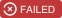# list-diff2

Diff two list

## Stats

StarsIssuesVersionUpdatedCreatedSize
list-diff2
17040.1.47 years ago7 years agolist-diff## Introduction

Diff two lists in time O(n). I The algorithm finding the minimal amount of moves is Levenshtein distance which is O(nm). This algorithm is not the best but is enougth for front-end DOM list manipulation.
This project is mostly influenced by virtual-dom
algorithm.

## Install

``\$ npm install list-diff2 --save``

## Usage

``````var diff = require("list-diff2")
var oldList = [{id: "a"}, {id: "b"}, {id: "c"}, {id: "d"}, {id: "e"}]
var newList = [{id: "c"}, {id: "a"}, {id: "b"}, {id: "e"}, {id: "f"}]

var moves = diff(oldList, newList, "id")
// `moves` is a sequence of actions (remove or insert):
// type 0 is removing, type 1 is inserting
// moves: [
//   {index: 3, type: 0},
//   {index: 0, type: 1, item: {id: "c"}},
//   {index: 0, type: 0},
//   {index: 4, type: 1, item: {id: "f"}}
//  ]

moves.forEach(function(move) {
if (move.type === 0) {
oldList.splice(move.index, 1) // type 0 is removing
} else {
oldList.splice(move.index, 0, move.item) // type 1 is inserting
}
})

// now `oldList` is equal to `newList`
// [{id: "c"}, {id: "a"}, {id: "b"}, {id: "e"}, {id: "f"}]
console.log(oldList) ``````